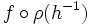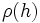External wreath product

This article describes a product notion for groups. See other related product notions for groups.

Definition

For an abstract group and a group of permutations

This definition uses the left action convention.

Let$G$ be any abstract group and$H$ be a group along with a homomorphism$\rho:H \to \operatorname{Sym}(S)$ for some set$S$ (in other words, we are given a permutation representation, or group action, of$H$). Then, the external wreath product of$G$ by$H$ (relative to$\rho$) is defined as the external semidirect product of the group$G^S$ by$H$, where an element$h \in H$ sends$f:S \to G$ to the function$f \circ \rho(h^{-1})$.

This wreath product is typically denoted as:$G \wr H$

The group$G$ is termed the base of the wreath product.

The wreath product can also be viewed as follows: The group$G^S$ is a direct product of$|S|$ copies of$G$, and the action of$h \in H$ is to permute the$|S|$ coordinates by the permutation$\rho(h)$.

There is a related notion of the restricted external wreath product. This is the same as above except that we replace functions$f:S \to G$ by finitely supported functions, i.e., functions for which the set of points for which the value is a non-identity element is finite.

For two abstract groups

Let$G,H$ be abstract groups. Then, the external wreath product of$G$ by$H$ is typically understood as the wreath product where the action of$H$ is taken to be the regular group action on itself as a set. In other words, the homomorphism$\rho$ is the natural embedding arising via Cayley's theorem. This wreath product is also termed the regular wreath product.

Equivalence with the internal wreath product

An internal wreath product is the equivalent to an external wreath product, except that here we start with the whole group, and find subgroups$G$ and$H$ such that the given group is an internal semidirect product of a direct product of copies of$G$ by$H$, acting via coordinate permutations only. A subgroup$G$ arising in this way is termed a base of a wreath product.

Examples

Base Acting group Wreath product as an abstract group GAP ID
cyclic group:Z2 cyclic group:Z2 acting regularly dihedral group:D8 (8,3)
cyclic group:Z2 cyclic group:Z3 acting regularly direct product of A4 and Z2 (24,13)
cyclic group:Z2 symmetric group:S3 acting as a symmetric group direct product of S4 and Z2 (48,48)
cyclic group:Z3 cyclic group:Z2 acting regularly direct product of S3 and Z3 (18,3)
cyclic group:Z4 cyclic group:Z2 acting regularly wreath product of Z4 and Z2 (32,11)
group of prime order group of prime order (same prime, acting regularly) wreath product of groups of order p

Facts

The wreath product of two groups of permutations

Suppose both$G$ and$H$ are being viewed along with specific group actions of each, i.e., they are both being viewed as groups of permutations, with$G$ acting on a set$A$ and$H$ acting on a set$S$. Then,$G \wr H$ acts naturally on two sets: the Cartesian product$A \times S$, and the function space$A^S$ of functions from$S$ to$A$.

Further information: Action of wreath product on Cartesian product, Action of wreath product on function space

Associativity of wreath product

The associativity law:$(G \wr H) \wr K \cong G \wr (H \wr K)$

holds with a suitable interpretation. For instance, if we are given the left side, we need to justify how$H \wr K$ is to be viewed as a group of permutations for the right side to make sense. Both$H$ and$K$ come as groups of permutations, and we equip$H \wr K$ with the induced action on the Cartesian product of the spaces they're acting on.

Further information: Wreath product is associative